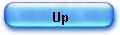HP35 inverse trigonometric algorithm

I will detail in this article the algorithm used in the HP 35 to compute inverse trigonometric functions: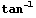,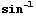and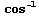.

A prefix key named "arc" is used, as shown in the user manual p.19 is used and than angle is returned in degrees.

I assume that my previous article on the HP35’s logarithm and direct trigonometric functions has been read, just to avoid repetition.

1) Vectoring with CORDIC.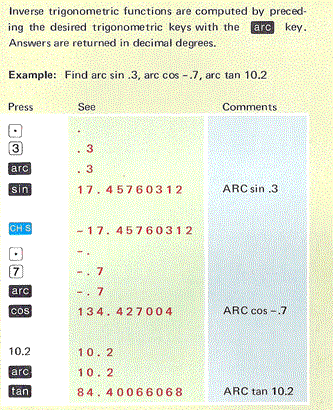In his original work paper published in 1959, Jack Volder covered in depth the subject for a "bit serial" computer.

The architecture described can work in two modes:

1) “rotation”,
- the input is an angle
θ, expressed in vector components (X0, Y0),
- rotations
αj tends to diminish the residual angle θα0α1 …- αj to zero,
- ouput is Yn and Xn, so that, Yn / Xn = tan(
θ).

2) “vectoring”,
- the input is a vector (X
0, Y0),
- rotations through whatever angle tends to align the resulting vector to the X axis, minimizing, thus, the Y component,
- the output being an angle  Z
n = tan-1 (Y0/X0).

There is here a common misunderstanding I want to clear away (based on a first reader’s feedback from my previous articles).

The Volder’s approach cannot be implemented as is, in a small calculator such as the HP 35.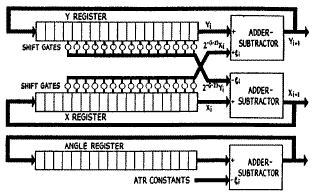In Volder’s own words: “CORDIC is an entire-transfer computer; it contains a special serial arithmetic unit consisting of three shift registers, three adder-subtractors, and special interconnections”.

The case of the HP 35 is different.
Ten years have passed and the firmware designer can use a full BCD arithmetic unit totally controlled by micro-program; that is why he preferred, there again, to follow the two pass pseudo-division & pseudo-multiplication approach designed by Meggitt (Cf.  ref 1 p. 216).

The Meggitt’s presentation does not follow the trigonometric classical scheme for the successive rotation formulas, but uses the complex numbers notation. This extension to the complex domain is just another paradigm.

For the direct trigonometric functions, Meggitt simply wrote (op. cit. p. 223, eq. 65):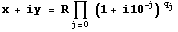where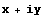is a complex number and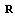a real.

Note that the multiplication of complex numbers is another way to express the composition of rotations. Then taking the complex logarithm of the equation, Meggitt could write (op. cit, p. 223, eq 64):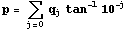and simply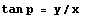(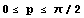).

Remember that the process was: by pseudo division (eq. 64) we can compute the set of exponents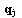and by pseudo multiplication (rotations) we simply formed equation 65.

For the inverse trigonometric function, we will indeed use the inverse process.

We start from a point in the plane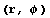and we rotate the vector clock wise to the x axis, minimizing thus the Y component and counting the number of rotations () for each angle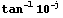.

Meggitt wrote: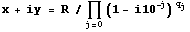(eq 21, p. 216)
which expresses clock wise (cf. the negative sign) rotation composition in the complex number notation.

Then he took the complex logarithm and the imaginary part, and had: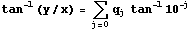(eq. 23, p. 216).

The process is there again in two parts: we’ll find numbersby pseudo-division first, and then we’ll compute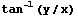by a pseudo-multiplication “using stored values for”.

The order of part1 and part2 are reversed; in part1 (compared to the direct trigonometric case) ; we process by to successive rotations in such way as to make the imaginary part of equation 21 as small as possible ; minimizing the Y component while keeping it positive (starting with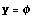and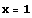).

The use of the pseudo-division process is quite similar as in the case of logarithm, but the pseudo divisor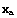is different (eq. 27, p.216, comp. eq.7, p.213).

At the end of part1, we have a set ofintegers as result.

The part2 of the process is straightforward, applying equation 23 exactly as done in the logarithm case.

To be quite clear, I’ll return to the trigonometric paradigm: in part 1, we make successive rotations to drive Y component close to zero. The angles of rotations are chosen to requires only shift and add to process () :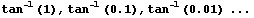The pseudo quotientmeans exactly how many times a rotation j is performed.

The formulas for rotations are the same as for direct trigonometric function except the sign: we rotate clock wise.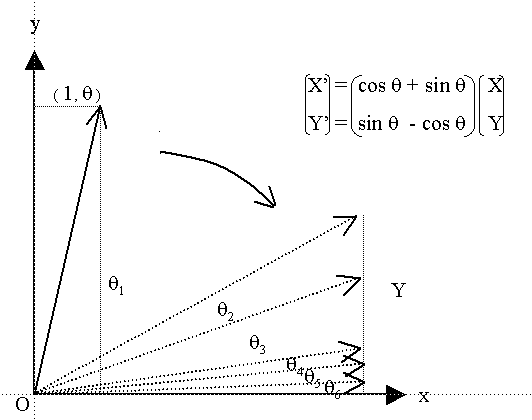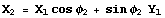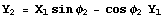Recurrence relations are also similar except for the sign: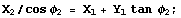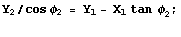It is in fact, very similar to the Volder’s original implementation, except for 3 points:

- constants are decimaland not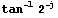,
- calculations are done in 2 passes, Cordic using simultaneously 3 adders-subtractors computes in 1 pass (of multiple rotations),
- Y component never gets negative.

2) Pseudo division: Cordic Rotations

The recurrence relations implemented are derived directly from the Cordic rotation equations given above: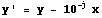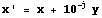Component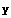is getting smaller all over the process, so to keep accuracy a small rewriting is convenient.
Introducing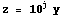, the previous relations now write: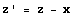(eq 1)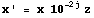(eq 2)

These are Meggitt’s equations n° 27 (p. 219) and that is exactly what is coded in the HP35’s ROM.

Here is the flow-chart of part 1 (pseudo division) ; the is simple to follow, provided that my previous articles have been read.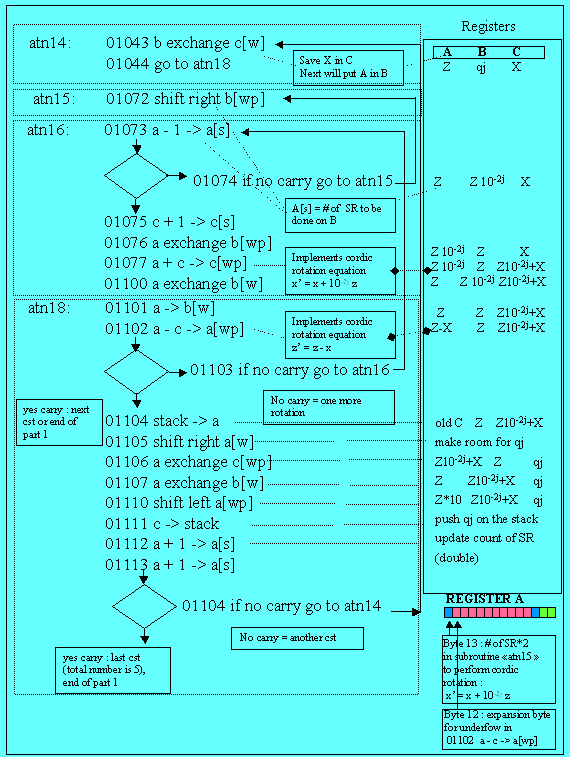I’m going to detail only one rotation: it is a repetitive process.

At [BP2] in the trace, we have made a first rotation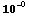.

The result is Z=19. (register B) and X=21. (register C).

As in the logarithm or tangent routines, byte 13 of register A is reserved for the shift right (SR) count and byte 12 for underflow expansion.

At [BP2A] address 0114, we have a carry trying to rotate by another 45° angle. So we are changing to constant tan-1(0.1); the code is at label atn14. We exchange B and C to use B for shifting operations, and we go to atn18.

Then, at [BP2B], the code implements equation 1 for the Cordic:
01102:             a - c -> a[wp]
thus the new Z is in A, the old in B and X is in C.

We test if this operation makes a carry, if yes we must change constant. If no, we get back to atn16.

At [BP2C], the routines atn15 and atn16 look like eca21 and eca22 of the logarithm algorithm since instruction a + c -> c[wp] which implements equation 2 of the Cordic () is done on shifted values. A difference is that the number of SR is doubled.

When B is correctly shifted to the right (depending on the parameter j), we perform equation 2, and update the count of qj in c[s]. That’s one loop.

If we do have a carry at address 01103, then that’s either the end of the loops for the constant (the current j) or the end of part 1.

We pull from the stack the previous value of C (set of qj) and we store the result we just got  for the current j, we mix them and store the whole result back onto the stack.

Last 2 things, we multiply by 10 the result Z, to save accuracy and we make a test to determine if it’s the end of part 1. For that, since we use only 5 constants, we just have to double the count of SR:
01112 a + 1 -> a[s]
01112 a + 1 -> a[s],
luckily we have to do it even if we there is no carry, to compute the next constant.

Further, in [BP3], we have finished the pseudo division process, in our example the77371 and the final components are: Y=0.0017656039685, in B (left aligned), and X=29.327744737 in C.

But there is a residual angle that has to be taken care of. Since it is very small, we’ll start the second part with ratio Z/X which is very close approximation of this small angle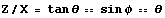.

For this calculation (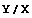) we call the division floating point routine. At [BP4] the division is done and the normalized result is: 0.602025134 (left aligned) and 6.02025134 10-5 in floating point.

The calculation trace of part 1 with Mathematica gives: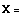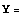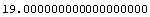etc.. down to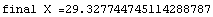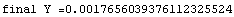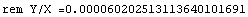(see the Mathematica trace and the Mathematica note book )

3) Pseudo multiplication

Now can process part 2 which looks like the process for logarithms (the constants loaded from ROM being different).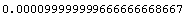arc tan(0.0001),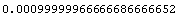arc tan(0.001),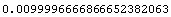arc tan(0.01),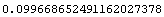arc tan(0.1),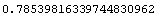arc tan(1) = pi/4,

and we start with the left aligned remainder Y/X and we form equation 23 (in fact we sum up all small angles).
At [BP5], the result (the angle) is in A normalized 1.520837931000.

We now have to convert this value from radians to degrees multiplying by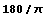; we first load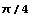, double it so we can divide the angle by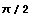[BP7] and finally multiply by 90 to have the result in degrees, which after normalization is = 87.137137594757

A=08713759476001  B=00200000000999  C=08713759476001

The result with Mathematica: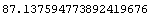4) Other inverse functions

The inverse functions for,are directly derived oncehas been generated, following the simple relations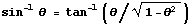and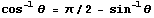:
- the code forbegins at address 01015, then mpy21, add10, sqt11, asn12, div11 to compute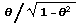and then  atn11 as above,
- the code forbegins at 01152 where we compute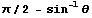(see for both cases).

Enjoy and please, give me feedback.
J. Laporte, Thursday, 22 December 2005.

revised 03/04/06

1) J.E. MEGGIT, Pseudo Division and Pseudo Multiplication processes, IBM Journal, Res & Dev, April 1062, 210-226. See trigonometry functions p.225.